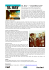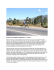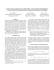# Two-ray model - UTH e

## Transcription

Two-ray model - UTH e
```Wireless signal propagation
(two ray model)
Thanasis Korakis
University of Thessaly
[email protected]
Two-ray model
●
Used when a single ground reflection
dominates the multipath effect
●
○ the LOS ray (the transmitted signal) and
○ a reflected ray, which is reflected off the ground, and
may interfere constructively or destructively
Why is this model so
important?
Free-space path loss model does not
consider interference.
Consider the problem of delivering wireless
coverage to an island using a wireless
backhaul from mainland
(this problem is based on a real scenario)
Low tide
Constructive interference
High-quality signal
High tide
Destructive interference
Very poor signal
Two-ray interference model
● Explains deep fades caused by multipath
interference
○ where they will occur, and how deep they will be
● Validated by measurements on many
different wireless systems
Two ray interference model
Two ray interference model
Distance traveled by direct ray:
Distance traveled by reflected ray:
Two ray interference model
Phase difference between rays:
Reflection coefficient at ground (depends on
material):
Two ray interference model
The received signal is the superposition of the
two rays. Using the free space path loss model:
the power of the two rays adds to:
Two ray interference model
Assume that delay spread is small compared to
signal bandwidth (narrowband assumption):
Then we can express the received power as:
What does the power look
like over distance?
Example on next slide based on real
measurements
Two ray model, large d
If we assume
d becomes
large, equal
gains, perfect
reflection:
Two ray model, large d
Then the phase difference becomes (using
Taylor expansion):
Two ray model, large d
and the expression for received power can be
simplified as:
Two ray model, as multi-slope model
● We saw that for large d received power Pr
decays according to
Pr = K P t / d 4
● For small d, two-ray model decays like freespace model, according to
Pr = K P t / d 2
(but two-ray model also include multipath)
Two ray model, as multi-slope model
Two ray model, as multi-slope model
What is considered large d?
● Power decays with d-4 when d > dc
dc = 4πhthr / λ
this is known as the critical distance, and
comes from the expression for phase difference
● Power decays with d-2 if d < dc
Simplified path loss models
● More generally: the main characteristics of
path loss (without multipath interference)
are captured by the expression:
Pr = K Pt [d0 / d]n
2≤n≤6
Includes free-space, two-ray, log-distance models
K factor (in detail)
● In free space & two ray models respectively:
K(free space) = Gt Gr λ2 / (4π)2 L
K(two ray) = Gt Gr ht2 hr2 / L
● Where
○
○
○
○
Gt and Gr are the Tx and Rx antenna gains
ht and hr are the Tx and Rx antenna heights
λ is the wavelength
L >=1 is the system loss factor (if not specified, L=1)
Log-distance PL model
PL = PL0 + 10nlog10( d / d0 )
Where:
○ PL0 is the Path loss in an indicative distance d0
○ n varies with propagation environments
Example 1
● Calculate free space & two ray path loss, given L=1, if :
○ frequency of signal is 2GHz
○ Tx and Rx antenna gains are 9dBi and 30dBi
○ Tx and Rx antenna heights are 15m and 1.5m
○ the distance Tx - Rx is 21Km
● 9dBi → 7.94 and 30dBi → 1000
○ since G(dBi)= 10 log10(G) (further details later)
● λ = c / f = 3x108 / 2x109 m = 0.15m
Example 1 (K factors)
● K(free space) = Gt Gr λ2 / (4π)2 L =
7.94 x 1000 x (0.15m)2 / (4π)2 x 1 = 1.13m2
● K(two ray) = Gt Gr ht2 hr2 / L =
7.94 x 1000 x (15m)2 x (1.5m)2 / 1 = 4019625m4
Example 1 (Path losses PL and dc)
● PL(free space) = 10log10(d2/K(free space) ) = 10log10((21x103)
2
/1.13) = 10log10(345.4x106) = 85.38dB
● PL(two ray) = 10log10(d4/K(two ray))=
10log10((21x103)4/4019625) = 10log10(0.048x1012) = 106.85
dB
● dc = 4π ht hr / λ = 4π x 15m x 1.5m / 0.15m = 1884m
○ d > d , so the two ray model predicts better
Multipath reception
○ the direct LOS radio wave, but also
○ a large number of reflected radio waves (not only
one like in two ray reflection model)
● In urban centers, LOS is often blocked by
obstacles and a collection of differently
delayed waves is all what is received
Assumes that a received multipath signal
consists of a (theoretically infinitely) large
number of reflected waves
This model has played a major role in our
understanding of mobile propagation, first
proposed by Lord Rayleigh in 1889
○ describing the resulting signal if many violinists
in an orchestra play in unison...
References
1. T. S. Rappaport, Wireless Communications:
Principle and Practice, 2nd ed. Singapore:
Pearson Education, Inc., 2002.
2. S. Haykin and M. Moher, Modern Wireless
Communications. Singapore: Pearson
Education, Inc., 2002.
```

### Find Homes for Sale in Columbia, SC at Ray Covington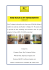Looking for homes for sale in Columbia, SC? At Ray Covington, we have a top team of experienced Real estate Agent and Realtor in Columbia, SC who are expertise in selling & purchasing of homes. Feel free to contact us at (803) 331-8833 at any time! Visit Us: http://raycovington.com/

### here - Crystallise!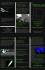### Sea Ray Overnighter 220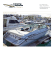### Ray J Crook Obituary web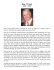### 17/63 George Street, BEENLEIGH, QLD, 4207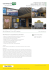### Shagreen Ray Leucoraja fullonica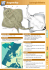### el ray_eng v2